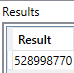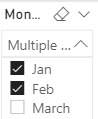Quite often I wanted to get the data a slicer quickly and easily where multiple items are selected.

I then wanted to use the slicer items to filter my measure.

The first thing that I did was to manually hard code the values to make sure that I was getting the correct values with the following measure below.

```EVALUATE
ROW (
"Result",
CALCULATE (
SUM ( MyTable[MTD Budget] ),
TREATAS ( { "Jan", "FEB" }, 'Date'[Month Name] )
)
)
```

And this was the result using DAX Studio.To show you what happens when the VALUES is being run in the context of the measure it returns the following as shown below with the measure.```CONCATENATEX (
VALUES ( 'Date'[Month Name]),
'Date'[Month Name],
","
)
```

The result is the following as shown below.Now to get this working with the Slicer Items I changed the measure to do the following below.

```CALCULATE (
SUM ( MyTable [MTD Budget] ),
TREATAS ( VALUES ( 'Date'[Month Name] ), 'Date'[Month Name] )
)
```

And once again I got the same result below, showing that it is getting back the correct values to filter.## Summary

In this blog post I have shown how to use TREATAS to capture items from your slicer and pass it through to your measure.